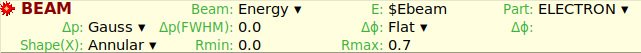# FWHM value of energy

What value has light speed in fluka. For example if I want set the value of FWHM for primary particle energy equals to 0.0015 GeV, what value of momentum FWHM I should set ?I want set the value of FWHM for primary particle energy equals to 0.0015 GeV. What value of momentum FWHM I should set ?

For instance, in the FLAIR manual section 3.6 you can find the values of the global variables.
http://flair.web.cern.ch/flair/manual/F3.6.html

In the flair section that @msabateg pointed, please pay attention to the the function

For example if in .inp file I write

#define deltaP -0.0015/c
BEAM \$Ebeam \$deltaP 0.7 0. -1.ELECTRON

is that mean that energy FWHM will be equal 1.5 MeV ?

Hi,

You can profit from the pre-defined function mentioned before in this post to ensure that the momentum spread will be properly converted from the energy spread you are interested on.

In order to do so, you can define a variable as:

#define deltaP : dT2dp(Ebeam,deltaE,m)

where Ebeam, deltaE and m are the input variables you need to specify.

Then, assign it to the Dp(FWHM) in the BEAM card or copy there the value you have obtained from this function.

Hope this helps you.

1 Like

Is here m the mass of particle in GeV ?

The mass of the particle should be in GeV/c2.

So if I write #define deltaP : dT2dp(0.028, 0.0015, Me/c^2) then I get right value for Dp when energy spread equals to 0.0015GeV ?

You just need to include the mass of the electron in GeV/c2 units, i.e.: 511e-6 GeV/c2. Therefore, in your function you should read:

dT2dp(0.028, 0.0015, 511e-6)

Sorry, I can’t understand. F3.6} Function evaluation here said that
c=299792458e2 cm/s and mass of electron in GeVs equals to Me=0.510998910e-3. How you obtain mass of the electron in GeV/c2 equals to 511e-6 GeV/c2 ?

The mass of the electron is certainly 511e-6 GeV/c2.
Nevertheless, in the unit system used in here, it appears as GeV (unit system where c=1) for simplicity not to have to include c=299792458e2 cm/s any time it appears.
So you can safely use Me in GeVs in the formula.

OK, thank you very much